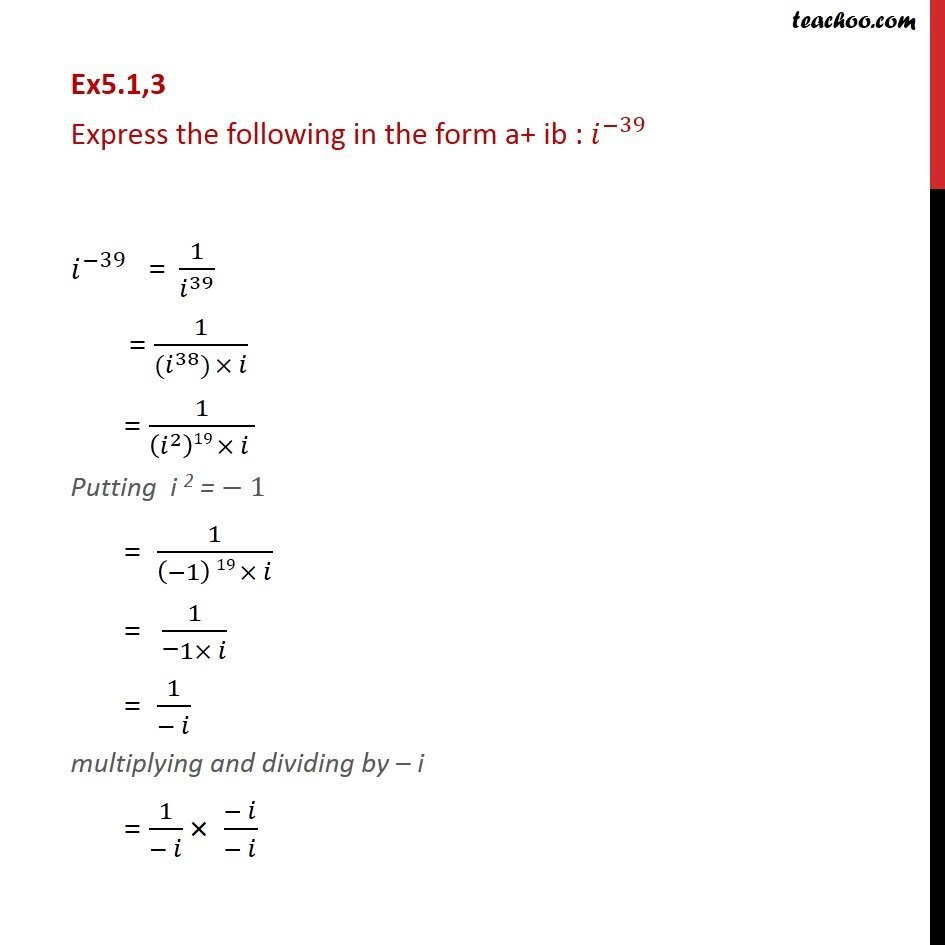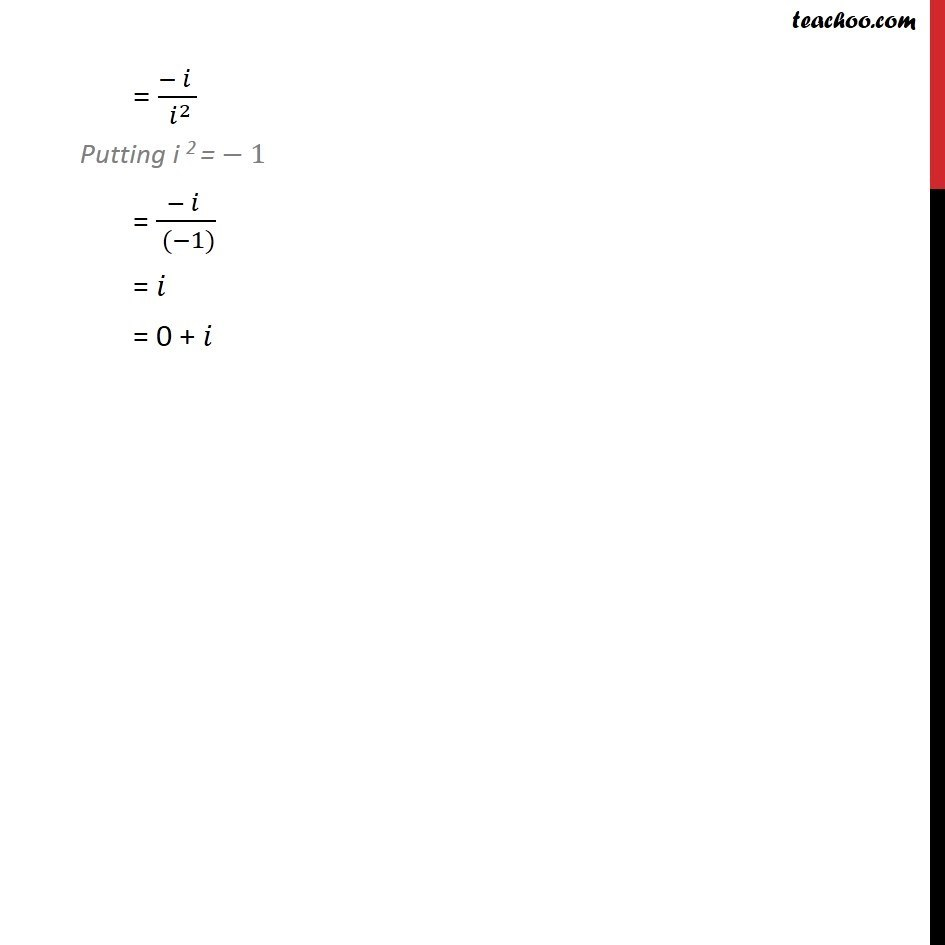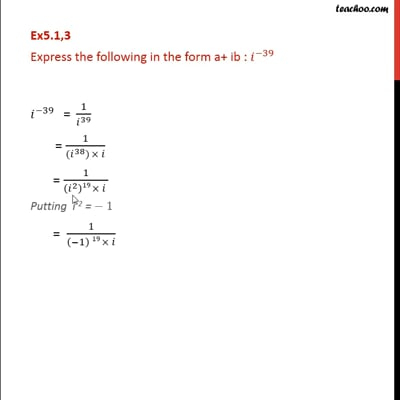Ex 5.1

Chapter 5 Class 11 Complex Numbers
Serial order wiseThis video is only available for Teachoo black users

Introducing your new favourite teacher - Teachoo Black, at only ₹83 per month

### Transcript

Ex5.1,3 Express the following in the form a+ ib : ^( 39) ^( 39) = 1/ ^39 = 1/( ( ^38) ) = 1/(( ^2 )19 ) Putting i 2 = 1 = 1/(( 1) 19 ) = 1/(" " 1 ) = 1/( ) multiplying and dividing by i = 1/( ) ( )/( ) = ( )/( ^2 ) Putting i 2 = 1 = ( )/( ( 1)) = = 0 +